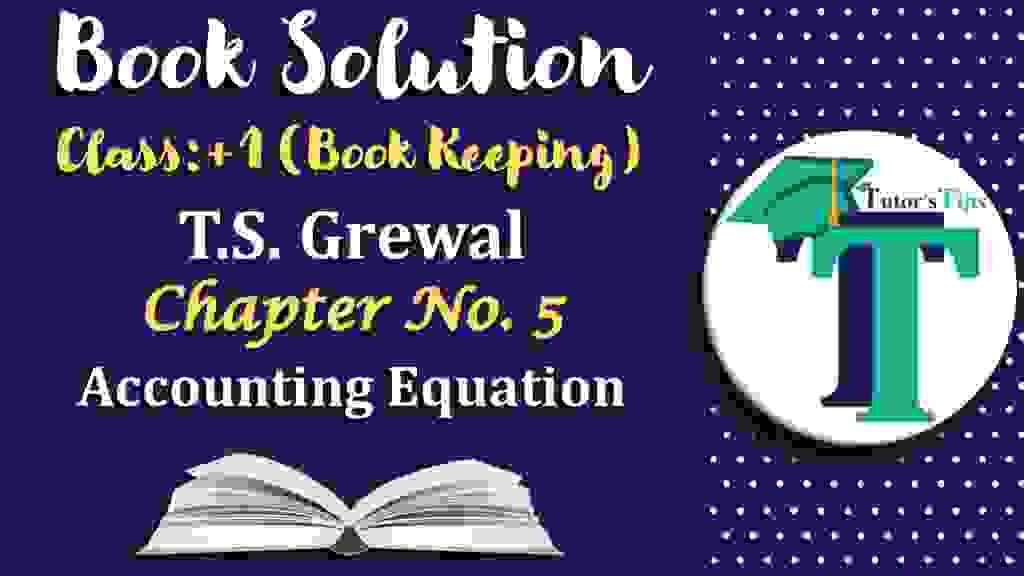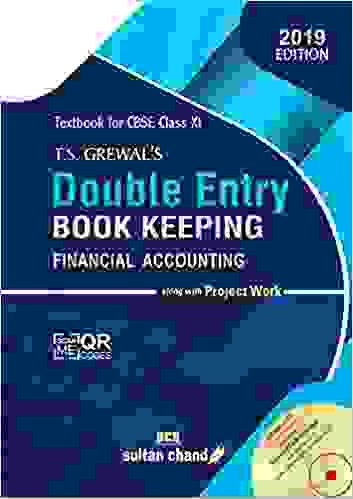# Chapter No. 5 – Accounting Equation – T.S. Grewal 11 Class – Book SolutionIn this article, we have to provide the Question wise solution to all Questions of a chapter of Accounting Equation – T.S. Grewal 11 Class – Book Solution But first of all, we have explained the meaning of Accounting Equation: –

## What is the Accounting Equation:

Accounting Equation is the basis of the double-entry system of accounting. The double-entry system means every business transaction has two aspects one is the Debit and another is the Credit. Example: – If we want to buy something like coke, pizza or burger we have to pay cash for these items, So in this transaction, we are receiving food and paying cash which means we are doing two jobs, that is why it is called double-entry system.

In the double-entry system, every Debit amount should be equal to the Credit amount Because :
The Business can receive cash or assets from two sources, one from an owner and another is the third party. When it received from an owner this is called Capital and when receiving from third parties then it is called liabilities.

So, in Accounting Equation, Cash or Assets always Equal to Capital and Liabilities of the Business.

## “Assets = Liabilities + Capital”

For more Explanation please check out the following link: –

What is Accounting Equation | Example

## Accounting Equation – T.S. Grewal 11 Class – Book Solution

Question wise solution of the all Questions of Accounting Equation – T.S. Grewal 11 Class – Book Solution are shown below: –T.S. Grewal’s Double Entry Book Keeping